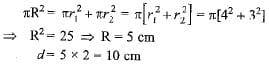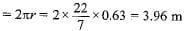Test: Areas Related to Circles - 1

# Test: Areas Related to Circles - 1

Test Description

## 10 Questions MCQ Test Mathematics (Maths) Class 10 | Test: Areas Related to Circles - 1

Test: Areas Related to Circles - 1 for Class 10 2022 is part of Mathematics (Maths) Class 10 preparation. The Test: Areas Related to Circles - 1 questions and answers have been prepared according to the Class 10 exam syllabus.The Test: Areas Related to Circles - 1 MCQs are made for Class 10 2022 Exam. Find important definitions, questions, notes, meanings, examples, exercises, MCQs and online tests for Test: Areas Related to Circles - 1 below.
Solutions of Test: Areas Related to Circles - 1 questions in English are available as part of our Mathematics (Maths) Class 10 for Class 10 & Test: Areas Related to Circles - 1 solutions in Hindi for Mathematics (Maths) Class 10 course. Download more important topics, notes, lectures and mock test series for Class 10 Exam by signing up for free. Attempt Test: Areas Related to Circles - 1 | 10 questions in 10 minutes | Mock test for Class 10 preparation | Free important questions MCQ to study Mathematics (Maths) Class 10 for Class 10 Exam | Download free PDF with solutions
 1 Crore+ students have signed up on EduRev. Have you?
Test: Areas Related to Circles - 1 - Question 1

### If the area of a circle is 154 cm2, then its perimeter is

Detailed Solution for Test: Areas Related to Circles - 1 - Question 1

Given area of circle=154
⇒area of circle=πr²
=22/7 ×7×7.
=154
so radius of the circle=7cm
perimeter of circle=2πr
=2 ×22/7×7
=44cm
⇒perimeter of the circle=44 cm.

Test: Areas Related to Circles - 1 - Question 2

### The radii of two circles are 4 cm and 3 cm respectively. The diameter of the circle having area equal to the sum of the areas of the two circles (in cm)

Detailed Solution for Test: Areas Related to Circles - 1 - Question 2Test: Areas Related to Circles - 1 - Question 3

### If the circumference of a circle and the perimeter of a square are equal, the

Test: Areas Related to Circles - 1 - Question 4

The perimeter (in cm) of a square circumscribing a circle of radius a cm, is

Detailed Solution for Test: Areas Related to Circles - 1 - Question 4

Side of a square circumscribing a circle of radius a cm = diameter of circle = 2a cm
∴ Perimeter of the square = 4 x 2a = 8a cm

Test: Areas Related to Circles - 1 - Question 5

The perimeter of a circle is equal to that of a square, then the ratio of their areas is​

Test: Areas Related to Circles - 1 - Question 6

If the area of a circle is numerically equal to twice its circumference, then the diameter of the circle is

Detailed Solution for Test: Areas Related to Circles - 1 - Question 6

πr2 - 2πr x 2 ⇒ r = 4 ⇒ 2r = 8 units

Test: Areas Related to Circles - 1 - Question 7

The area of the circle that can be inscribed in a square of side 6 cm is

Test: Areas Related to Circles - 1 - Question 8

The diameter of a wheel is 1.26 m. The distance travelled in 500 revolutions is

Detailed Solution for Test: Areas Related to Circles - 1 - Question 8

Radius of the wheel = 1.26/2 = 0.63 m
Distance travelled in one revolution∴ Distance travelled in 500 revolutions
= 500 x 3.96 = 1980 m

Test: Areas Related to Circles - 1 - Question 9

The radius of a circle whose circumference is equal to the sum of the circumferences of the two circles of diameters 36 cm and 20 cm is​

Detailed Solution for Test: Areas Related to Circles - 1 - Question 9

Diameter of first circle = d1 = 36 cm
Diameter of second circle = d2 = 20 cm
∴ Circumference of first circle = πd1 = 36π cm
Circumference of second circle = πd2 = 20π cm
Now, we are given that,
Circumference of circle = Circumference of first circle + Circumference of second circle
πD = πd1 + πd2
⇒ πD = 36π + 20π
⇒ πD = 56π ⇒ D = 56
⇒ Radius = 56/2 = 28 cm

Test: Areas Related to Circles - 1 - Question 10

If the sum of the circumferences of two circles with radii R1 and R2 is equal to the circumference of a circle of radius R, then

Detailed Solution for Test: Areas Related to Circles - 1 - Question 10

2π R1 + 2πR2 = 2πR
⇒ R1 + R2 = R.

## Mathematics (Maths) Class 10

53 videos|369 docs|138 tests
 Use Code STAYHOME200 and get INR 200 additional OFF Use Coupon Code
Information about Test: Areas Related to Circles - 1 Page
In this test you can find the Exam questions for Test: Areas Related to Circles - 1 solved & explained in the simplest way possible. Besides giving Questions and answers for Test: Areas Related to Circles - 1, EduRev gives you an ample number of Online tests for practice

## Mathematics (Maths) Class 10

53 videos|369 docs|138 tests